# 彻底理解红黑树及JavaJDK1.8TreeMap源码分析

## 2. 节点称呼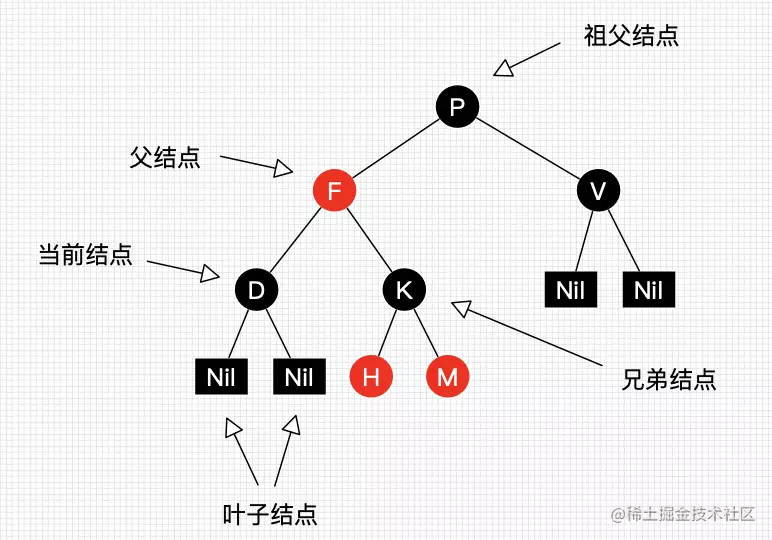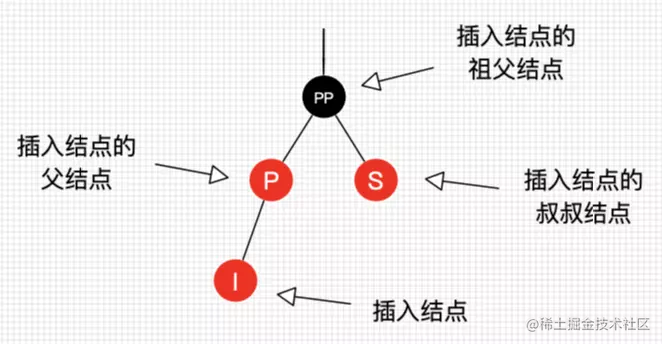## 3. 性质

• 每个节点要么是黑色，要么是红色。

• 根节点一定是黑色。

• 每个叶子节点（nil或null）都是黑色的。

• 每个红节点的两个子节点一定是黑色的。（不可以同时存在两个相连的红结点，即：红节点的父结点与子结点都是黑的）

• 从任意节点出发到每个叶子节点的路径都包含相同个数的黑色节点。

``````* 如果一个结点存在黑子结点，那么该结点肯定有两个子结点。

* 黑色完美平衡。
复制代码``````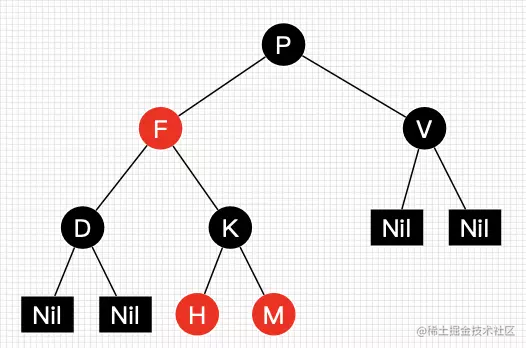## 4. 红黑树的自平衡

### 4.1 左旋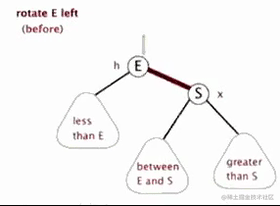### 4.2 右旋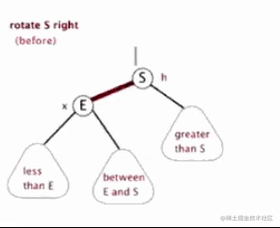## 5. 红黑树的查找

• 从根节点开始查找，为空就返回null，为当前值就返回，否则继续向下查找。
• 如果当前节点的key为要查找的节点的key，那么直接返回当前值。
• 如果当前节点的key大于要查找的节点的key，那么继续向当前节点的左子节点查找。
• 如果当前节点的key小于要查找的节点的key，那么继续向当前节点的右子节点查找。

## 6. 红黑树的插入

### 6.1 查找插入位置

• 插入节点的颜色肯定为红色。因为插入节点为黑色，就会破坏黑色完美平衡，使得到叶子节点的黑色数+1，而红色不会破坏。
• 基本与红黑树的查找相同：

• 如果当前节点为null，则返回当前节点的父节点进行插入。
• 如果当前节点的key等与插入节点的key，则更新当前节点的value。
• 如果当前节点的key大于插入节点的key，则继续向当前节点的左子节点继续查找。
• 如果当前节点的key小于插入节点的key，则继续向当前节点的右子节点继续查找。

### 6.2 插入的自平衡

• 将父节点左旋
• 将指针指向父结点

• 将父节点变为黑色
• 将祖父结点变为红色
• 将祖父结点右旋
• 将指针指向祖父结点

• 当前结点是根结点直接变为黑色
• 当前结点不是根结点
• 将父节点与叔叔节点变为黑色
• 将祖父结点变为红色
• 将指针指向祖父结点

JDK1.8中插入自平衡的源码实现：

``````private void fixAfterInsertion(Entry<K,V> x) {
x.color = RED;

while (x != null && x != root && x.parent.color == RED) {
// 插入的父节点是左子节点
if (parentOf(x) == leftOf(parentOf(parentOf(x)))) {
// y是插入节点的祖父节点的右子节点（叔叔节点）
Entry<K,V> y = rightOf(parentOf(parentOf(x)));
// y是红色
if (colorOf(y) == RED) {
// 变色处理
setColor(parentOf(x), BLACK);
setColor(y, BLACK);
setColor(parentOf(parentOf(x)), RED);
// 指针指向插入节点的祖父节点
x = parentOf(parentOf(x));
} else {
// y是黑色的
// 插入节点是是父节点的右子节点
if (x == rightOf(parentOf(x))) {
// 父节点左旋
x = parentOf(x);
rotateLeft(x);
}
// 插入节点是是父节点的左节点
setColor(parentOf(x), BLACK);
setColor(parentOf(parentOf(x)), RED);
// 祖父节点右旋
rotateRight(parentOf(parentOf(x)));
}
} else {
// 插入的父节点是右子节点
// y是插入节点的祖父节点的左子节点（叔叔节点）
Entry<K,V> y = leftOf(parentOf(parentOf(x)));
// y是红色
if (colorOf(y) == RED) {
// 变色处理
setColor(parentOf(x), BLACK);
setColor(y, BLACK);
setColor(parentOf(parentOf(x)), RED);
// 指针指向插入节点的祖父节点
x = parentOf(parentOf(x));
} else {
// 插入节点是是父节点的左子节点
if (x == leftOf(parentOf(x))) {
// 父亲节点右旋
x = parentOf(x);
rotateRight(x);
}
// 插入节点是是父节点的右子节点
setColor(parentOf(x), BLACK);
setColor(parentOf(parentOf(x)), RED);
// 祖父节点左旋
rotateLeft(parentOf(parentOf(x)));
}
}
}
root.color = BLACK;
}

## 7. 红黑树删除

### 7.1 查找删除位置

• 从根节点开始，如果根节点为空，则删除在根节点，否则根节点为当前节点。
• 如果当前节点为null，则返回当前节点的父节点进行插入。
• 如果当前节点的key等与删除节点的key，则找到当前节点。
• 如果当前节点的key大于删除节点的key，则继续向当前节点的左子节点继续查找。
• 如果当前节点的key小于删除节点的key，则继续向当前节点的右子节点继续查找。

### 7.2 删除结点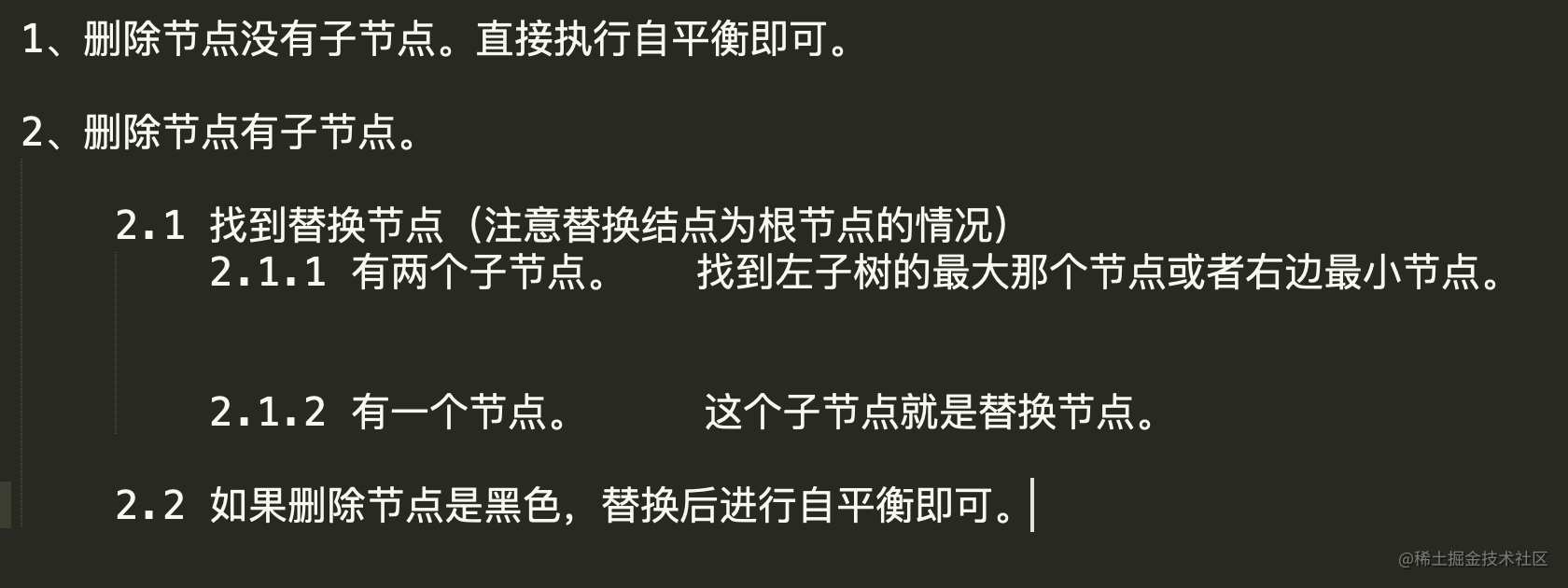JDK1.8中TreeMap删除可能性源代码实现：

``````private void deleteEntry(Entry<K,V> p) {
modCount++;
size--;

// If strictly internal, copy successor's element to p and then make p
// point to successor.
// 如果删除节点有两个子节点
if (p.left != null && p.right != null) {
// 找到替代节点（很简单，自己看TreeMap源码）
Entry<K,V> s = successor(p);
p.key = s.key;
p.value = s.value;
p = s;
} // p has 2 children

// Start fixup at replacement node, if it exists.
// 如果有一个替换节点
Entry<K,V> replacement = (p.left != null ? p.left : p.right);

// 如果存在替换节点
if (replacement != null) {
replacement.parent = p.parent;
if (p.parent == null)
root = replacement;
else if (p == p.parent.left)
p.parent.left  = replacement;
else
p.parent.right = replacement;

// Null out links so they are OK to use by fixAfterDeletion.
p.left = p.right = p.parent = null;

// Fix replacement
if (p.color == BLACK)
fixAfterDeletion(replacement);
}
// 如果删除节点是根节点
else if (p.parent == null) { // return if we are the only node.
root = null;
} else {
// 没有子节点
if (p.color == BLACK)
fixAfterDeletion(p);

if (p.parent != null) {
if (p == p.parent.left)
p.parent.left = null;
else if (p == p.parent.right)
p.parent.right = null;
p.parent = null;
}
}
}

### 7.3 删除后的自平衡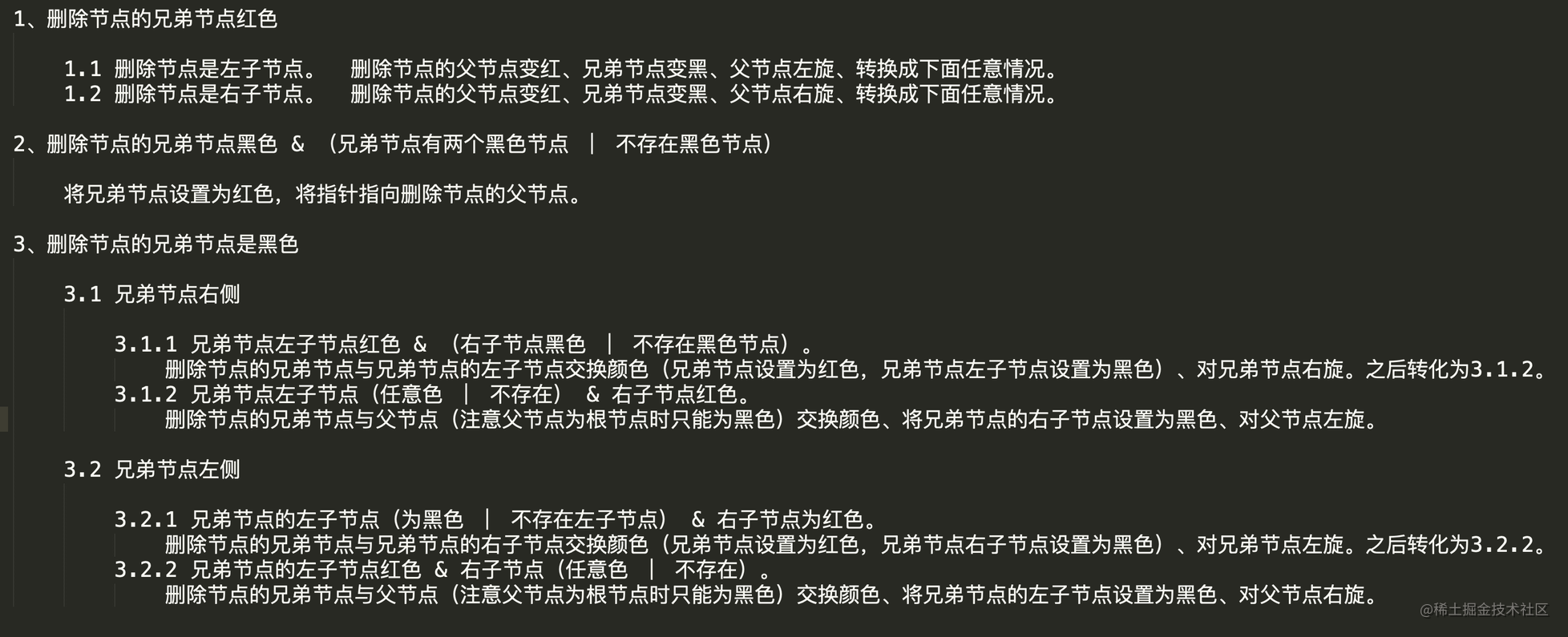JDK1.8中TreeMap删除自平衡源代码实现：

``````private void fixAfterDeletion(Entry<K,V> x) {
while (x != root && colorOf(x) == BLACK) {
// 删除节点是左子节点
if (x == leftOf(parentOf(x))) {
// sib是删除节点父节点的右子节点（兄弟节点）
Entry<K,V> sib = rightOf(parentOf(x));
// 兄弟节点是红色
if (colorOf(sib) == RED) {
// 情况1.1处理
setColor(sib, BLACK);
setColor(parentOf(x), RED);
rotateLeft(parentOf(x));
sib = rightOf(parentOf(x));
}
// sib兄弟节点有两个黑色的子节点，情况2处理
if (colorOf(leftOf(sib))  == BLACK &&
colorOf(rightOf(sib)) == BLACK) {
// 变色
setColor(sib, RED);
// 指针指向删除节点的父节点
x = parentOf(x);
} else {
// 兄弟节点的右子节点是黑色
if (colorOf(rightOf(sib)) == BLACK) {
// 情况3.1.1处理
setColor(leftOf(sib), BLACK);
setColor(sib, RED);
rotateRight(sib);
sib = rightOf(parentOf(x));
}
// 情况3.1.2处理
setColor(sib, colorOf(parentOf(x)));
setColor(parentOf(x), BLACK);
setColor(rightOf(sib), BLACK);
rotateLeft(parentOf(x));
// 跳出循环
x = root;
}
} else { // symmetric
// 删除节点是右子节点
// sib是删除节点父节点的左子节点（兄弟节点）
Entry<K,V> sib = leftOf(parentOf(x));
// 兄弟节点是红色
if (colorOf(sib) == RED) {
// 情况1.2处理
setColor(sib, BLACK);
setColor(parentOf(x), RED);
rotateRight(parentOf(x));
sib = leftOf(parentOf(x));
}
// sib兄弟节点有两个黑色的子节点，情况2处理
if (colorOf(rightOf(sib)) == BLACK &&
colorOf(leftOf(sib)) == BLACK) {
// 变色
setColor(sib, RED);
// 指针指向删除节点的父节点
x = parentOf(x);
} else {
// 兄弟节点的左子节点是黑色
if (colorOf(leftOf(sib)) == BLACK) {
// 情况3.2.1处理
setColor(rightOf(sib), BLACK);
setColor(sib, RED);
rotateLeft(sib);
sib = leftOf(parentOf(x));
}
// 情况3.2.2处理
setColor(sib, colorOf(parentOf(x)));
setColor(parentOf(x), BLACK);
setColor(leftOf(sib), BLACK);
rotateRight(parentOf(x));
// 跳出循环
x = root;
}
}
}

setColor(x, BLACK);
}